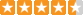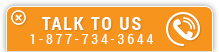# Structural Vibration Frequency Analysis of Beams

Course Number: S-3001
Credit: 3 PDH
Subject Matter Expert: C. Sundararajan, Ph.D., MASCE, FASME
Price: \$89.85 Purchase using Reward Tokens. Details41 reviews
Overview

#### In Structural Vibration Frequency Analysis of Beams, you'll learn ...

• Compute the fundamental natural frequency of beams carrying one or more concentrated weights
• Compute the fundamental natural frequency of beams carrying uniformly distributed weight and concentrated weight
• Determine the effect axial loading on the fundamental frequency

#### OverviewPreview a portion of this course before purchasing it.

Credit: 3 PDH

Length: 22 pages

Fundamental natural frequency is an important parameter in structural dynamics analysis and applications. A number of simple formulas for the computation of the fundamental natural frequency of beam vibration under a variety of boundary conditions and loading are presented. Some are approximate formulas and some are exact. An estimate of the error is given for the approximate formulas whenever possible. The formulas presented here are simple enough that the fundamental frequency could be obtained by hand calculation without recourse to computers or finite element analysis. The formulas are demonstrated with nine worked out numerical examples.

#### Specific Knowledge or Skill Obtained

This course teaches the following specific knowledge and skills:

• Compute the fundamental natural frequency of beams carrying one or more concentrated weights
• Compute the fundamental natural frequency of beams carrying uniformly distributed weight and concentrated weight
• Determine the effect axial loading on the fundamental frequency

#### Certificate of Completion

You will be able to immediately print a certificate of completion after passing a multiple-choice quiz consisting of 20 questions. PDH credits are not awarded until the course is completed and quiz is passed.

Board Acceptance
 This course is applicable to professional engineers in: Alabama (P.E.) Alaska (P.E.) Arkansas (P.E.) Delaware (P.E.) Florida (P.E. Area of Practice) Georgia (P.E.) Idaho (P.E.) Illinois (P.E.) Illinois (S.E.) Indiana (P.E.) Iowa (P.E.) Kansas (P.E.) Kentucky (P.E.) Louisiana (P.E.) Maine (P.E.) Maryland (P.E.) Michigan (P.E.) Minnesota (P.E.) Mississippi (P.E.) Missouri (P.E.) Montana (P.E.) Nebraska (P.E.) Nevada (P.E.) New Hampshire (P.E.) New Jersey (P.E.) New Mexico (P.E.) New York (P.E.) North Carolina (P.E.) North Dakota (P.E.) Ohio (P.E. Self-Paced) Oklahoma (P.E.) Oregon (P.E.) Pennsylvania (P.E.) South Carolina (P.E.) South Dakota (P.E.) Tennessee (P.E.) Texas (P.E.) Utah (P.E.) Vermont (P.E.) Virginia (P.E.) West Virginia (P.E.) Wisconsin (P.E.) Wyoming (P.E.)
Reviews (41)
More DetailsPreview a portion of this course before purchasing it.

Credit: 3 PDH

Length: 22 pages